# IB DP Physics: HL复习笔记10.2.3 Potential Gradient & Difference

• An electric field can be defined in terms of the variation of electric potential at different points in the field:

The electric field at a particular point is equal to the negative gradient of a potential-distance graph at that point

• The potential gradient is defined by the equipotential lines
• These demonstrate the electric potential in an electric field and are always drawn perpendicular to the field lines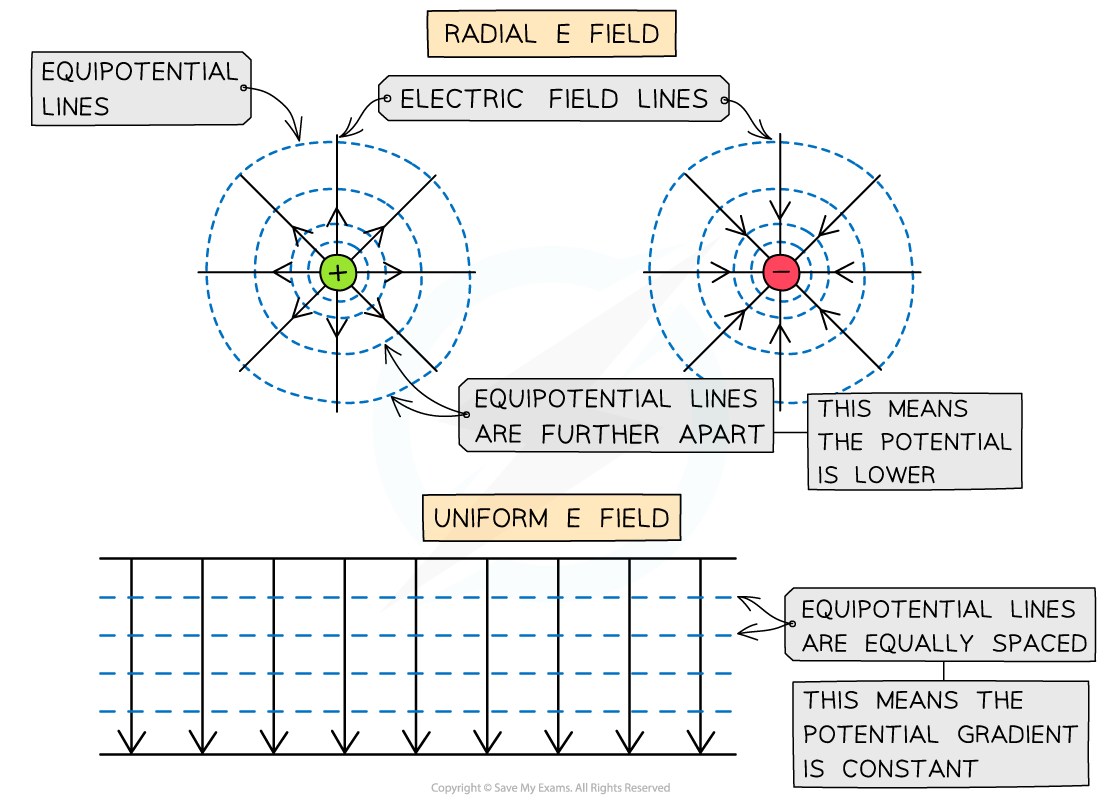Equipotential lines around a radial field or uniform field are perpendicular to the electric field lines

• Equipotential lines are lines of equal electric potential
• Around a radial field, the equipotential lines are represented by concentric circles around the charge with increasing radius
• The equipotential lines become further away from each other
• In a uniform electric field, the equipotential lines are equally spaced
• The potential gradient in an electric field is defined as:

The rate of change of electric potential with respect to displacement in the direction of the field

• The electric field strength is equivalent to this, except with a negative sign:
•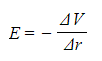Where:
• E = electric field strength (V m-1)
• ΔV = change in potential (V)
• Δr = displacement in the direction of the field (m)
• The minus sign is important to obtain an attractive field around a negative charge and a repulsive field around a positive charge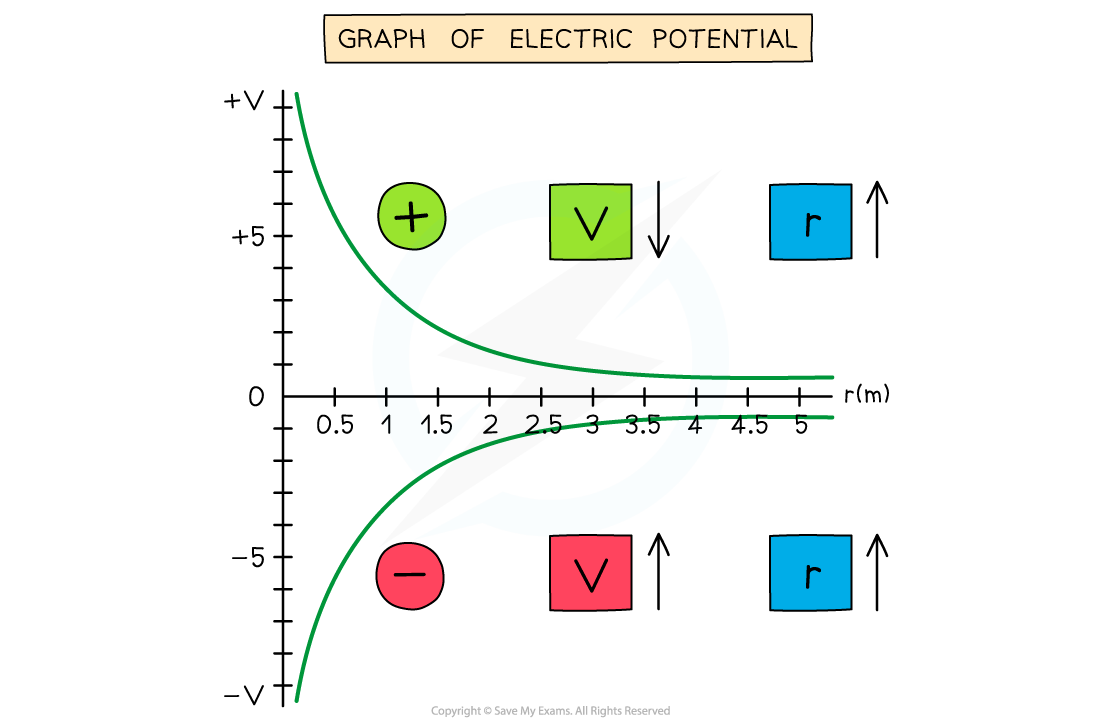The electric potential around a positive charge decreases with distance and increases with distance around a negative charge

• The electric potential changes according to the charge creating the potential as the distance r increases from the centre:
• If the charge is positive, the potential decreases with distance
• If the charge is negative, the potential increases with distance
• This is because the test charge is positive

• A gravitational field can be defined in terms of the variation of gravitational potential at different points in the field:

The gravitational field at a particular point is equal to the negative gradient of a potential-distance graph at that point

• The potential gradient is defined by the equipotential lines
• These demonstrate the gravitational potential in a gravitational field and are always drawn perpendicular to the field lines
• The potential gradient in a gravitational field is defined as:

The rate of change of gravitational potential with respect to displacement in the direction of the field

• Gravitational field strength, g and the gravitational potential, V can be graphically represented against the distance from the centre of a planet, r
•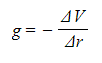Where:
• g = gravitational field strength (N kg-1)
• ΔV = change in gravitational potential (J kg-1)
• Δr = distance from the centre of a point mass (m)
• The graph of V against r for a planet is: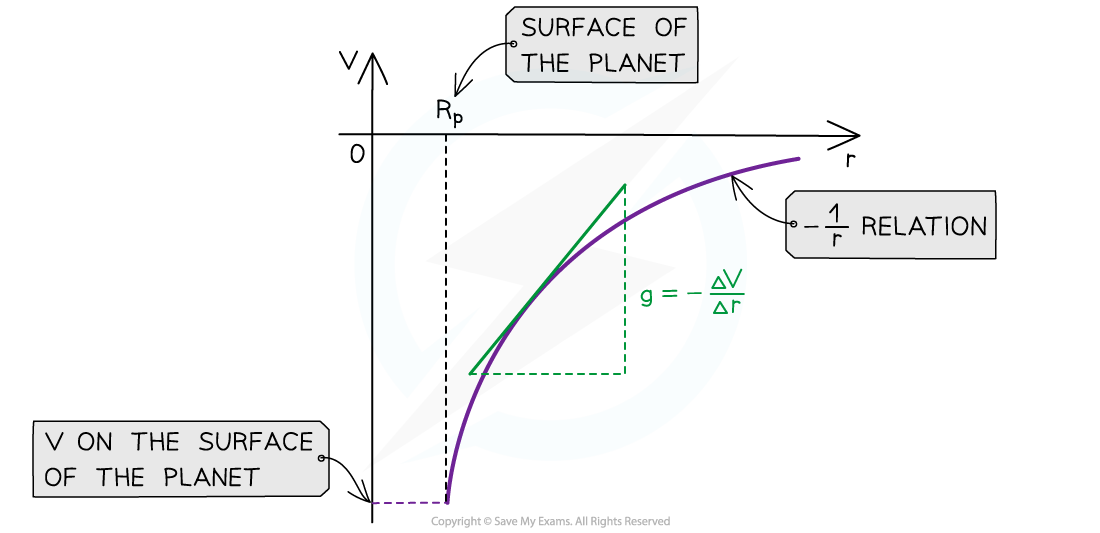The gravitational potential and distance graphs follows a -1/r relation
• The key features of this graph are:
• The values for V are all negative
• As r increases, V against r follows a -1/r relation
• The gradient of the graph at any particular point is the value of g at that point
• The graph has a shallow increase as r increases
• To calculate g, draw a tangent to the graph at that point and calculate the gradient of the tangent
• This is a graphical representation of the equation: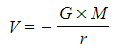where G and M are constant

#### Worked Example

Determine the change in gravitational potential when travelling from 3 Earth radii (from Earth’s centre) to the surface of the Earth.

Assume that the mass of the Earth is 5.97 × 1024 kg and the radius of the Earth is 6.38 × 106 m

Step 1: List the known quantities
• Earth’s mass, ME = 5.97 × 1024 kg
• Radius of the Earth, rE = 6.38 × 106 m
• Initial distance, r1 = 3 × rE = 3 × (6.38 × 106) m = 1.914 × 107 m
• Final distance, r2 = 1 × rE = 6.38 × 106 m
• Gravitational constant, G = 6.67 × 10−11 m3 kg−1 s−2
Step 2: Write down the equation for potential difference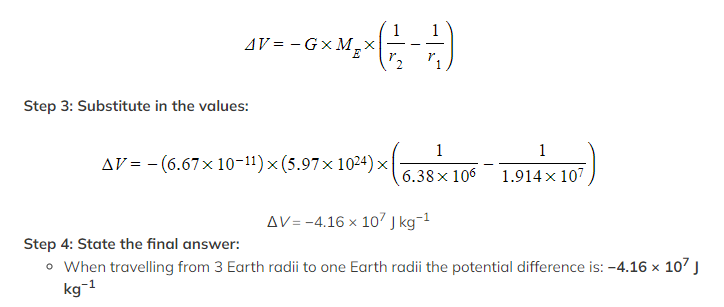Exam Tip
One way to remember whether the electric potential increases or decreases with respect to the distance from the charge is by the direction of the electric field lines. The potential always decreases in the same direction as the field lines and vice versa.

### Potential Difference

• The potential difference is defined as:

The work done by moving a positive test charge from one point to another in an electric field

• The units for potential difference are:
• Joules per Coulomb for electrostatic potential difference
• Joules per Kilogram for gravitational potential difference
• This can be related to the concept of equipotentials where the movement of a small test mass or positive test charge from one equipotential to another visually represents a potential difference
• Further, potential difference also occurs across electrical components which is also known as voltage

#### Uniform Electric Field Strength

• The magnitude of the electric field strength in a uniform field between two charged parallel plates is defined as:
•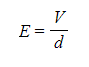Where:
• E = electric field strength (V m-1)
• V = potential difference between the plates (V)
• d = separation between the plates (m)
• Note: the electric field strength is now also defined by the units V m-1
• The equation shows:
• The greater the voltage between the plates, the stronger the field
• The greater the separation between the plates, the weaker the field
• This equation cannot be used to find the electric field strength around a point charge (since this would be a radial field)
• The direction of the electric field is from the plate connected to the positive terminal of the cell to the plate connected to the negative terminalThe E field strength between two charged parallel plates is the ratio of the potential difference and separation of the plates

• Note: if one of the parallel plates is earthed, it has a voltage of 0 V

#### Derivation of Electric Field Strength Between Plates

• When two points in an electric field have a different potential, there is a potential difference between them
• To move a charge across that potential difference, work needs to be done
• Two parallel plates with a potential difference ΔV across them create a uniform electric field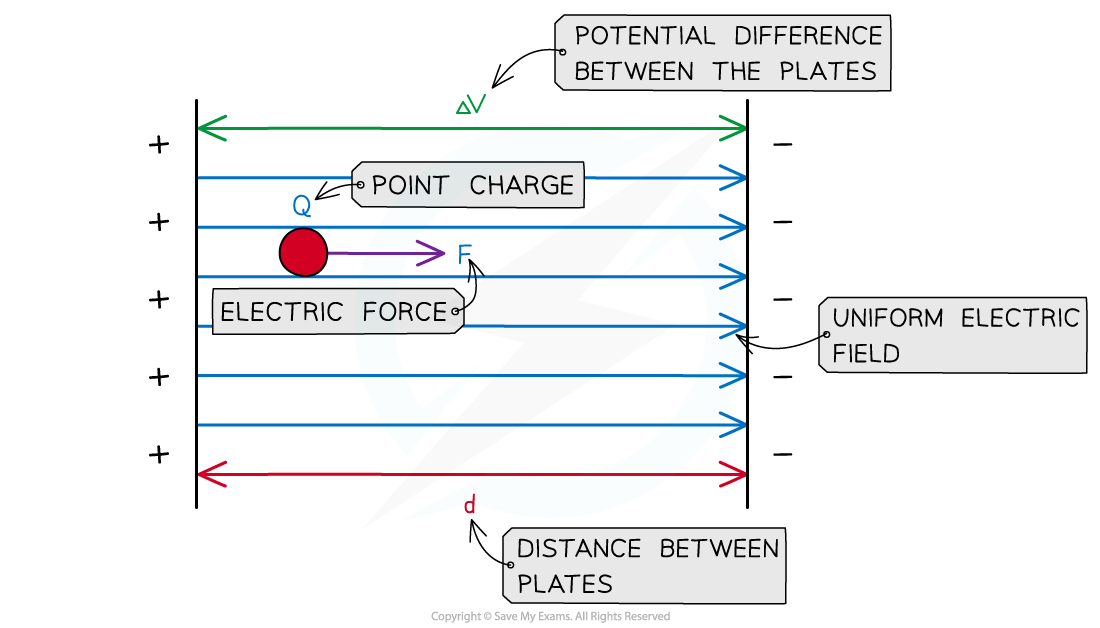The work done on the charge depends on the electric force and the distance between the plates

• Potential difference is defined as the energy, W, transferred per unit charge, Q, this can also be written as: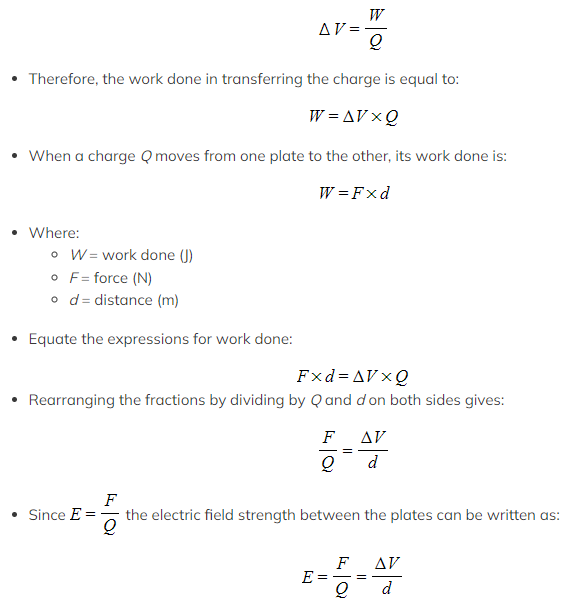Worked Example

Two parallel metal plates are separated by 3.5 cm and have a potential difference of 7.9 kV.

Calculate the magnitude of the electric force acting on a stationary charged particle between the plates that has a charge of 2.6 × 10-15 C.

Step 1: Write down the known values
• Potential difference, V = 7.9 kV = 7.9 × 103 V
• Distance between plates, d = 3.5 cm = 3.5 × 10−2 m
• Charge, Q = 2.6 × 10−15 C
Step 2: Write down the equation for the electric field strength between the parallel plates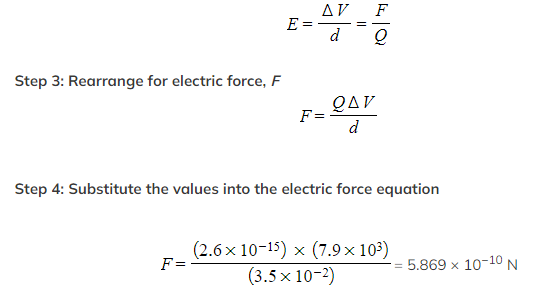Step 5: State the final answer
• The magnitude of the electric force acting on this charged particle is 5.9 × 10−10 N

#### Exam Tip